## If Value is Greater Than A Certain Value

IF function is frequently used in Excel worksheet to return you expect “true value” or “false value” based on the logical test result. If you want to see if a value in one cell is greater than a specific value, you can use IF function to build a formula with logical test argument is “value>certain value”, IF function can return either true result or false result based on the test result.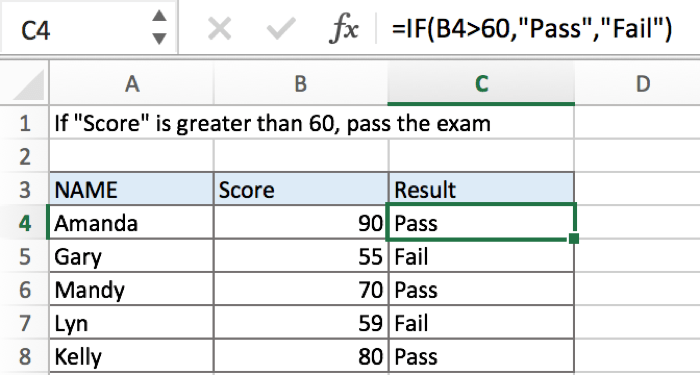In above example, in column “Score”, if cell value is greater than 60, then “Pass” is saved in “Result”; if cell value is not reached 60, “Fail” is saved in “Result”.

## FORMULA

To test if cell equals a certain text string, the generic formula is:

=IF(A1>X,”true value”,”false value”)

Formula in this example:

=IF(B4>60,”Pass”,”Fail”)

## EXPLANATION

In this example, for a student, if his score is greater than 60, that means he passed the exam, and we will add comment “Pass” in “Result” column for students who pass the exam; on the other side, for those who failed exam with a score lower than 60, we will add comment “Fail” in result. To return proper value based on a comparison, IF function can handle case like this effectively.

IF function allows you to create a logical comparison between your value and reference value (for example “A1>0”), and set true value and false value what you expect to return as test results. IF function returns one of the two results based on logical comparison result.

Syntax: IF(logica_test,[value_if_true],[value_if_false])

To test if B4 is greater than 60, we can directly create a logical comparison B4>60. There is no need to add double quotes “” to quote number 60 like B4>”60”, if you test B4=”ABC” then “ABC” should be enclosed into double quotes.

In this example, value in B4 is 90, 90>60 is true, so logical test B4>60 returns true.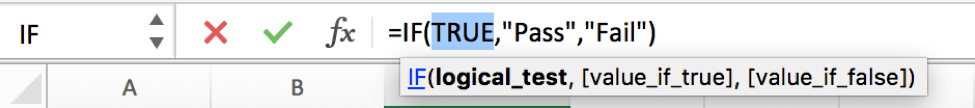As the result is True, IF function retrieves the value from “value if true” argument, in this case it is a text “Pass”, so “Pass” is displayed in C5. But for B5, B5 is 55 which a value lower than 60, so IF retrieves False value “Fail” and return “Fail” as result.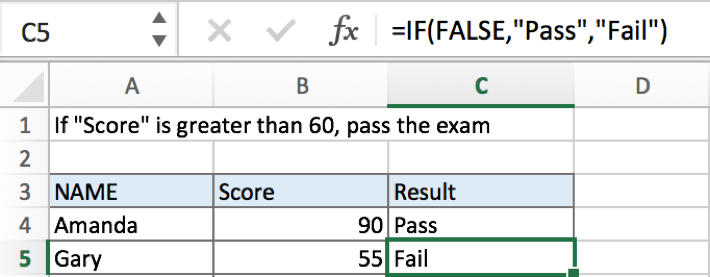In IF, true value and false value can be set as empty string “”, a text, a number or a formula, you can set what you expect to these two values, see examples below:

a.If value is not greater than 60, returns nothing by IF function.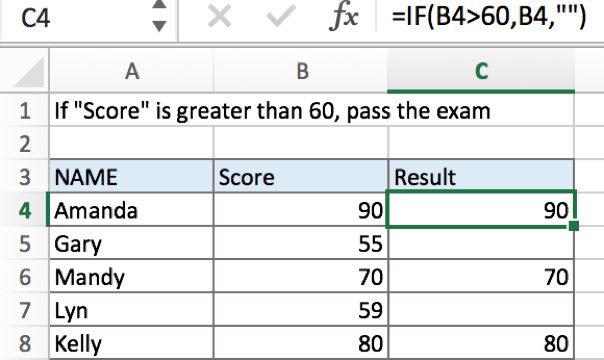b.If value is greater than 60, returns the value by IF function.c.If value is greater than 60, returns the value which is equal to “value-60” by IF function.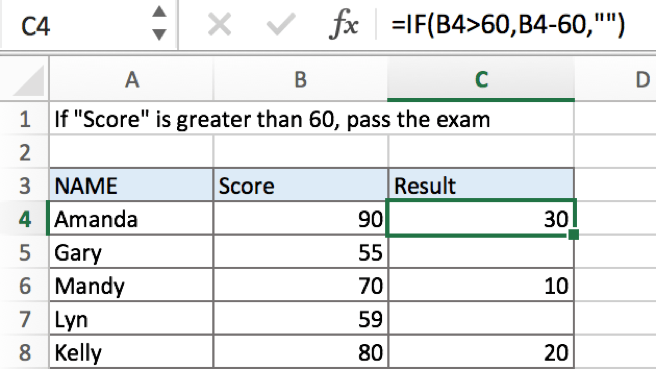### Related Functions

• Excel IF function
The Excel IF function perform a logical test to return one value if the condition is TRUE and return another value if the condition is FALSE. The IF function is a build-in function in Microsoft Excel and it is categorized as a Logical Function.The syntax of the IF function is as below:= IF (condition, [true_value], [false_value])….

## Excel IF function check if the cell is blank or not-blank

If you want to check the value in one cell if it is blank or empty cell, then you can use IF function in combination with ISBLANK function or logical operator (equal to) in excel.

## Excel IF function check if the cell is blank

Let’s see the generic IF formula with ISBLANK function and Logical operator = as follows:

```=IF(ISBLANK(B1),"PASS","FAILED")
=IF(B1="","PASS","FAILED")```

In the above two IF formulas, it will return “PASS” if Cell B1 is blank, Otherwise returns “FAILED”.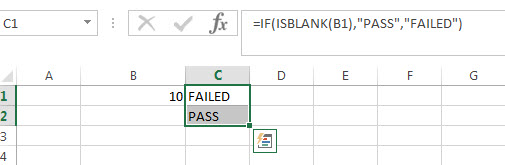## Excel IF function check if the cell is non-blank

If you want to check the value in one cell if it is not blank or not empty cell, then you can use IF function in combination with ISBLANK function or logical operator (not equal to) in excel.

let’s see the generic IF formula with ISBLANK function and Logical operator <> as follows:

```=IF(ISBLANK(B1)=FALSE,"PASS","FAILED")
=IF(B1<>"","PASS","FAILED")```

In the above two IF formulas, it will return “PASS” if Cell B1 is not blank, otherwise returns “FAILED”.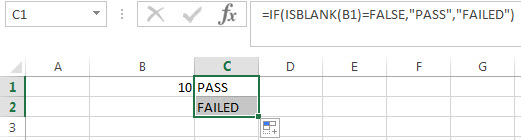Let’s write a nested if function in combining with AND function and ISBLANK function to achieve the following test criteria.

IF cell A1 is greater than or equal to 10, then add to C1.

If Cell B1 is greather than or equal to 10, then subtract from C1.

If both A1 and b1 are blank cells, then equals to C1.

Based on the above logic, we can write down the following excel nested if formula (statement):

`=IF(A1>=0,C1+A1, IF(B1>=0,C1-B1, IF(AND(ISBLANK(A1)=TRUE, ISBLANK(B1)=TRUE),C1,"")))`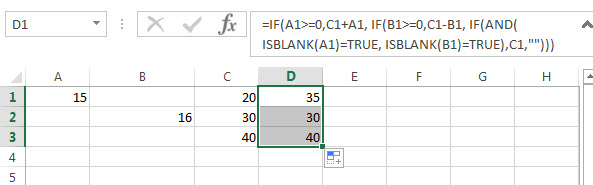### Related Formulas

• Excel IF formula with operator : greater than,less than
Now we can use the above logical test with greater than operator. Let’s see the below generic if formula with greater than operator:=IF(A1>10,”excelhow.net”,”google”) …

• Excel IF function with text values
If you want to write an IF formula for text values in combining with the below two logical operators in excel, such as: “equal to” or “not equal to”…
• Excel IF Function With Numbers
If you want to check if a cell values is between two values or checking for the range of numbers or multiple values in cells, at this time, we need to use AND or OR logical function in combination with the logical operator and IF function…
• Excel IF function with Dates
If you have a list of dates and then want to compare to these dates with a specified date to check if those dates is greater than or less than that specified date. …

### Related Functions

• Excel AND function
The Excel AND function returns TRUE if all of arguments are TRUE, and it returns FALSE if any of arguments are FALSE.The syntax of the AND function is as below:= AND (condition1,[condition2],…) …
• Excel IF function
The Excel IF function perform a logical test to return one value if the condition is TRUE and return another value if the condition is FALSE….
• Excel nested if function
The nested IF function is formed by multiple if statements within one Excel if function. This excel nested if statement makes it possible for a single formula to take multiple actions…
• Excel ISBLANK function
The Excel ISBLANK function returns TRUE if the value is blank or null.The syntax of the ISBLANK function is as below:= ISBLANK (value)…

## Excel IF formula with “Equal to” logical operators

The “Equal to” logical operator can be used to compare the below data types, such as: text string, numbers, dates, Booleans.  This section will guide you how to use “equal to” logical operator in excel IF formula with text string value and dates value.

 Formula example Description =IF(A1=B1,“TRUE”,”FALSE”) Returns TRUE if a value in cell A1 is equal to the value in cell B1, FALSE if not. =IF(A1=”excehow”,”TRUE”,”FALSE”) Returns TRUE if a value in cell A1 is equal to “excelhow”, FALSE if not. =IF(A1=TRUE,”TRUE”,”FALSE”) Returns TRUE if a value in cell A1 is equal to TRUE, FALSE if not. =IF(A1=(B1/5),”TRUE”,”FALSE”) Returns TRUE if a value in cell A1 is equal to the quotient of the division of B1 by 5, FALSE if not.

## The Generic IF formula using “equal to” logical operators with text values

Let’ see the below formula:

`=IF(B1=”excelhow”,True_result, False_result)`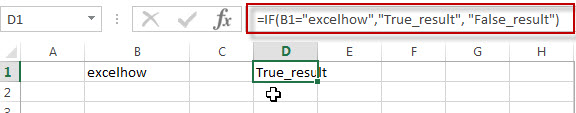If you want to do some special action when a value in a cell equal to a certain value, then you can use IF function with logical operator to constructing a condition statement. If the condition statement is true, then do some action.

The logical test is B1=”excelhow”in the above formula, and if cell B1 is equal to the string “excelhow”, then returns “True_results“； If FALSE, return “False_result”.

We want to set the value in Cell D1, we only need to take action when the condition test is TRUE. Here, we will add“True_result” string to Cell D1 when the value in cell B1 is “excelhow”. If the value in cell B1 is not “excelhow”, then we will add “False_result” string into Cell D1.

## The Generic IF formula using “equal to “   logical operators with dates value

If the cells B1 and B2 contain the date value “10/29/2018”, if we use logic test B1=”10/29/2018” in the formula =B1=”10/29/2018”, you will get the FALSE result. Or if you use logic test B1=B2 in formula =B1=B2, then it will return TRUE.

So if you want to check one value in cells, you must use DATEVALUE function to warp a date to compare with a specific date value. For example: =B1=DATEVALUE(“10/29/2018”), at this time, it will return TRUE.

Now we can write a Generic IF formula using “equal to” with date value as follows:

`=IF(B1= DATEVALUE(“10/29/2018”),”excelhow”,”google”)`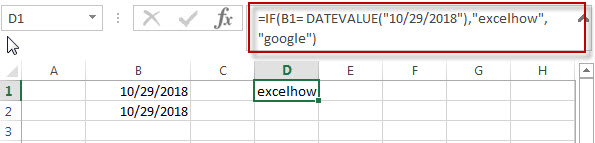## The Generic IF formula using “equal to “   logical operators with Boolean values and numbers

The Boolean value of TRUE Values equates to 1 and The FALSE value is equal to 0.

When writing a logical test using “equal to” operator that compares a Boolean value and a number. Assuming that using the logical test “1=True”, you will get the False result. it’s not our expected result.  At this time, you can use the double minus sign in the front of a Boolean value in a logical test. For example:”1=–TRUE

`=IF(A1=--B1,"excelhow.net","google.com")`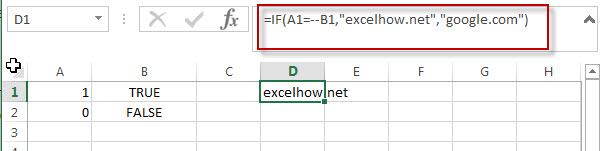### Related Formulas

• Excel IF formula with operator : greater than,less than
how to use if function with “greater than”,  “greater than or equal to”, ”less than” and “less than or equal to” logical operators in excel.Let’s see the below generic if formula with greater than operator:=IF(A1>10,”excelhow.net”,”google”) …

### Related Functions

• Excel IF function
The Excel IF function perform a logical test to return one value if the condition is TRUE and return another value if the condition is FALSE….
• Excel nested if function
The nested IF function is formed by multiple if statements within one Excel if function. This excel nested if statement makes it possible for a single formula to take multiple actions…